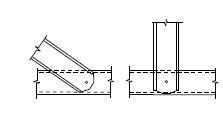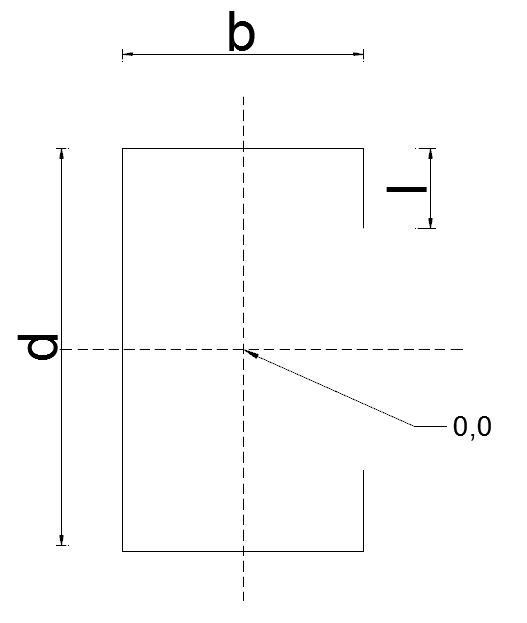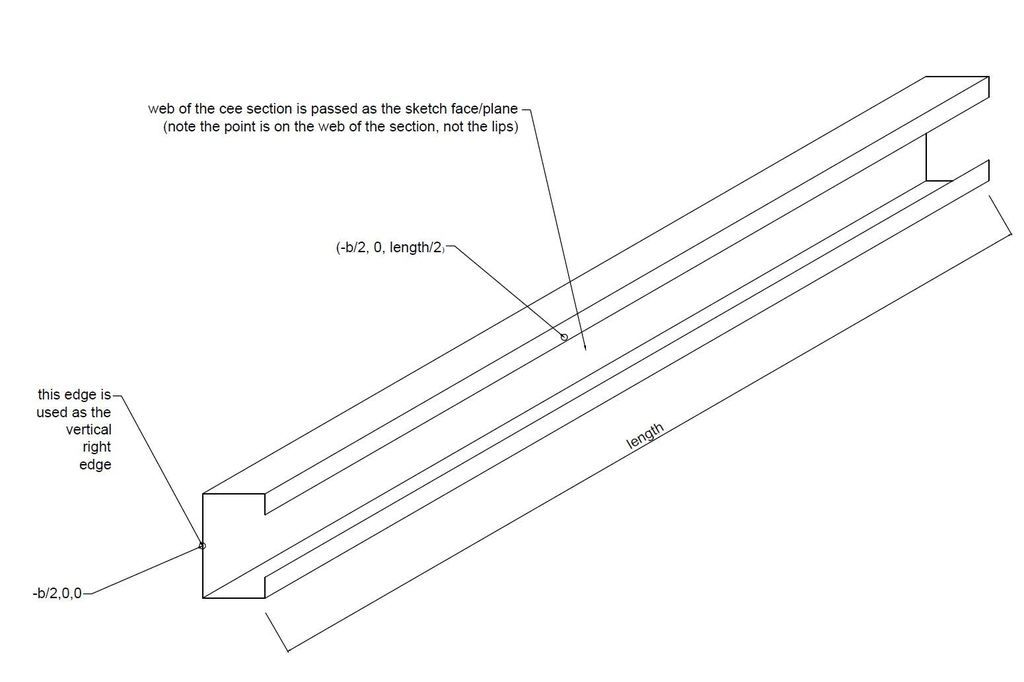# CutExtrude error Abaqus

Hi all,

I'm trying to create a full-scale truss for a static loading simulation using Abaqus.
I wanted to use a Python script to do this, but I am not very experienced with this language or the Abaqus methods.

The members forming the truss are cee sections. However, at the ends of the cee sections I want to create an extruded cut

like so:[/URL]]

However, when I try to use the CutExtrude command, Abaqus keeps giving me an "Invalid sketch plane" error even though I'm sure that the face I'm putting in the CutExtrude method is correct.

Here is the picture of the cross section of my cee section[/URL]]

Here are the co-ordinates I used to find the faces and the edges for the CutExtrude method[/URL]]

Here is my Python Code

from abaqus import *
from abaqusConstants import *
import regionToolset

session.viewports['Viewport: 1'].setValues(displayedObject=None)

#--------------------------------------------------------------------------------
#Create the model

mdb.models.changeKey(fromName='Model-1', toName='Malaysian Truss')

import sketch
import partition
import part
TrussModel = mdb.models['Malaysian Truss']

#-----------------------------------------------------------------------------------
#Create the parts

#web member 1 of the truss assembly. Web members being the diagonal members between the top and bottom chord members of a truss

Web_cross_section_sketch= TrussModel.ConstrainedSketch(name='Member Cross Section', sheetSize=100)
d=100
b=60
l=20
length1=1600

Web_cross_section_sketch.Line(point1=(b/2,d/2-l), point2=(b/2,d/2))
Web_cross_section_sketch.Line(point1=(b/2,d/2), point2=(-b/2,d/2))
Web_cross_section_sketch.Line(point1=(-b/2,d/2), point2=(-b/2,-d/2))
Web_cross_section_sketch.Line(point1=(-b/2,-d/2), point2=(b/2,-d/2))
Web_cross_section_sketch.Line(point1=(b/2,-d/2), point2=(b/2,-d/2+l))

Truss_web_part_1=TrussModel.Part(name='Truss Web Member 1', dimensionality=THREE_D, type=DEFORMABLE_BODY)

Truss_web_part_1.BaseShellExtrude(sketch=Web_cross_section_sketch, depth= length1)

#web member 2

length2=1800

Truss_web_part_2=TrussModel.Part(name='Truss Web Member 2', dimensionality=THREE_D, type=DEFORMABLE_BODY)

Truss_web_part_2.BaseShellExtrude(sketch=Web_cross_section_sketch, depth= length2)

#apex gusset plate

Apex_gusset_sketch= TrussModel.ConstrainedSketch(name='Apex Gusset', sheetSize=100)
bapex=600
dapex= 100
hapex= 400

Apex_gusset_sketch.Line(point1=(-bapex/2,0), point2=(-bapex/2,dapex))
Apex_gusset_sketch.Line(point1=(-bapex/2,dapex), point2=(0,hapex))
Apex_gusset_sketch.Line(point1=(0,hapex), point2=(bapex/2,dapex))
Apex_gusset_sketch.Line(point1=(bapex/2,dapex), point2=(bapex/2,0))
Apex_gusset_sketch.Line(point1=(bapex/2,0), point2=(-bapex/2,0))

Truss_apex_gusset=TrussModel.Part(name='Apex Gusset', dimensionality=THREE_D, type=DEFORMABLE_BODY)
Truss_apex_gusset.BaseShell(sketch=Apex_gusset_sketch)

#Create the cuts at the end of the web members

cut_depth=40
cut_width=20
web_face_point_web=(-b/2,0,length1/2)
member_draw_edge_1_point=(-b/2,0,0)

web_face_1_web=Truss_web_part_1.faces.findAt((web_face_point_web,))
member_draw_edge_1=Truss_web_part_1.edges.findAt((member_draw_edge_1_point,))

Member_cut_transform=Truss_web_part_1.MakeSketchTransform(sketchPlane=web_face_1_web, sketchUpEdge=member_draw_edge_1,sketchPlaneSide=SIDE1, sketchOrientation=RIGHT,origin=(-b/2,0,length1/2))

Web_end_cut_sketch_TL=TrussModel.ConstrainedSketch(name='Member End Cut', sheetSize=100, transform=Member_cut_transform)
#Web_end_cut_sketch_TL.rectangle(point1=(0,0),point2=(10,10))
Web_end_cut_sketch_TL.Line(point1=(-length1/2,d/2),point2=(-length1/2+cut_depth,d/2))
Web_end_cut_sketch_TL.Line(point1=(-length1/2+cut_depth,d/2), point2=(-length1/2,d/2-cut_width))
Web_end_cut_sketch_TL.Line(point1=(-length1/2,d/2-cut_width), point2=(-length1/2,d/2))

Web_end_cut_sketch_TL=TrussModel.ConstrainedSketch(name='Member End Cut', sheetSize=100, transform=Member_cut_transform)
Truss_web_part_1.CutExtrude(sketchPlane=web_face_1_web, sketchUpEdge=member_draw_edge_1,sketchOrientation=RIGHT, sketchPlaneSide=SIDE1, sketch=Web_end_cut_sketch_TL)

Any help that you could provide with this issue would be greatly appreciated.

thank you very much,
Victor

Free Tags: